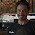## Binary simplified!

Let's do this simple. 0 means off and 1 means on.
Now, look around, you will see everything working in this pattern.
It's day, 1; it's night, 0. Switch on, 1; switch off, 0.

Computers take input in power. So, power on is 1 whereas power off is 0. In digital Electronics 1 stands for high voltage and 0 for low.
Suppose, I have 2 inputs. Both of them are set to 0. Do you think my machine will work? No. If one is 0 and the other is 1. Will my machine work now? No, because collective power is still low. It will start if and only if both the inputs are 1.

That's how it works! Two inputs get me 4 outputs and using this everything is derived.
0 is 00
1 is 01
2 is 10
3 is 11

And when I get 3 inputs this is what I'll have.
0 is 000
1 is 001
2 is 010
3 is 011
4 is 100
5 is 101
6 is 110
7 is 111

Have fun!

1.